Next: Transconductance Model Up: Bipolar Junction Transistors Previous: Small-Signal Models

## Ideal and Perfect Bipolar Transistor Models

By ignoring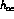and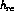we can define a simplified AC model for the transistor (perfect transistor) that is independent of the circuit configuration: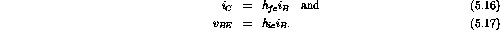There is no PN voltage drop in these equations since it is a DC effect.

Since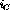is typically 100 times larger than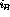we can make the approximation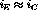. An ideal transistor is defined such that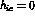and hence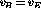. When the effects of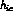cannot be ignored, we can use the perfect transistor model as described by the two equations above. On a circuit diagramcan be added directly to the ideal transistor symbol.

The ideal transistor model has the following two working rules:

1. The base and emitter are at the same AC voltage (). They differ only by a constant DC potential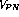.
2. The collector current is equal to the emitter current and proportional to the base current (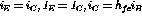and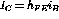).

Doug Gingrich
Tue Jul 13 16:55:15 EDT 1999